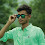Please visit, subscribe and share 10 Minutes Lectures in Computer Science

## How to test for conflict serializability of a schedule? Testing conflict serializability using precedence graph, How to draw a precedence graph, What is precedence graph, testing conflict serializability examples

### Testing for conflict serializability

Whether a schedule is conflict serializable or not can be determined using a directed graph called precedence graph.
What is precedence graph? It is a directed graph (also called as serialization graph) G with set of nodes N (T1, T2, T3, …, Tn) and set of directed edges E (E1, E2, ..., Em).

• the set of nodes N = the transactions of a schedule S that are trying to access same data item.
• the set of edges E = the set of directed links between two nodes (transactions).

### How to construct precedence graph?

Nodes
The total numbers of transactions in a schedule S will be the number of nodes in the precedence graph. For example, if the schedule S has three transactions, then there will be three nodes in the precedence graph.
Edges
An edge is a directed link between two different transactions Ti and Tj and will look something like Ti à Tj. Only tricky point here is that the link head (arrow) will be included if one of the following is True;
• Create a directed edge Ti → Tj, if Tj reads the value of an item Q written by Ti. [Read-Write conflict]
• Create a directed edge Ti → Tj, if Tj writes a value into an item Q after it has been read by Ti. [Write-Read conflict]
• Create a directed edge Ti → Tj, if Tj writes a value into an item Q after it has been written by Ti. [Write-Write conflict]

Example 1
Let us construct the precedence graph for the schedule S given below;
 Time Transaction T1 Transaction T2 1 2 3 4 5 6 7 8 9 10 11 12 13 read(A); A := A – 50; write(A); read(B); B := B + 50; write(B); read(A); temp := A * 0.1; A := A – temp; write(A); read(B); B := B + temp; write(B);
We have two transactions, hence two nodes namely, T1 and T2 [see figure 1(a)].Figure 1 - Construction of precedence graph for example 1
A Write(A) instruction (instruction 3) of T1 comes before Read(A) instruction (instruction 7) of T2. That is, T2 reads A that was written by T1. Here, instruction of T1 happens first then T2. So include an edge from T1 to T2 (Green color) [see figure 1(b)].
A Write(B) instruction (instruction 6) of T1 comes before Read(B) instruction (instruction 11) of T2. That is, T2 reads B that was written by T1. Here, instruction of T1 happens first then T2. So include an edge from T1 to T2 (Blue color) [see figure 1(c)].
In the precedence graph, both the edges are directed towards T2. Hence, the final graph is the one given in figure 1(d).
How do we conclude whether schedule S is conflict serializable or not?
Very simple.

• If your precedence graph has no cycle, then the schedule is conflict serializable.
• If your precedence graph has formed a cycle, then the schedule is not conflict serializable.
Our precedence graph above has no cycle in it. Hence, schedule S is conflict serializable and the serial order is T1-T2. That is, concurrent schedule S is equivalent to a serial schedule T1-T2.

Example 2
Is the following schedule S serializable or not?
 Time Transaction T1 Transaction T2 1 2 3 4 5 6 7 8 9 10 11 12 read(A); A := A – 50; write(A); read(B); B := B + 50; write(B); read(A); temp := A * 0.1; A := A – temp; write(A); read(B); B := B + temp; write(B);
We have two transactions in schedule S, so two nodes in the precedence graph as follows [see figure 2(a)];Figure 2 - Construction of precedence graph for example 2
T1 reads A before T2 writes A. Hence include an edge from T1 to T2 (Green color) [figure 2(b)].
T2 reads B before T1 writes B. Hence include an edge from T2 to T1 (Red color) [figure 2(c)].
Observe from the figure that a cycle has formed. Hence the schedule S is not conflict serializable.
[You can include edges for the other conflicts also. An edge from T2 to T1 for write A of T2 comes before write A of T1. Another edge from T1 to T2 for write B of T1 occurs before write B of T2. Anyhow, we have included the edges already. So, no need to specify for multiple times]

*******************

1.This comment has been removed by a blog administrator.

2.This comment has been removed by a blog administrator.

3.thank you you

4.Draw the precedence graph for the following expression:

S1: x:=0
S2: x:=x+1
S3: y:=2
S4: z:=y
S5: x:x+2
S6: y:=x+z
S7: z:=4

1.What are S1, S2, and so on?

## Featured Content

### Multiple choice questions in Natural Language Processing Home

MCQ in Natural Language Processing, Quiz questions with answers in NLP, Top interview questions in NLP with answers Multiple Choice Que...

data recovery